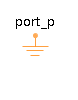User-defined - MapleSim Help

User-defined

User-defined tire model is used to apply input contact patch forces/moments onto the tire hubThe User-defined tire model, unlike the Linear, Fiala, Calspan and Pacejka tire models, allows the forces generated at the contact patch to be defined externally and passed to the model as inputs. The signal input ports on the component represent the user-specified forces and moments along and about the ISO unit vectors.

For more information, see the diagram below and the Standard Tire help page.Note: The $\mathrm{hubFrame}$ port of the User-defined tire component must be connected directly to the $\mathrm{hubFrame}$ port on a tire body component, as shown in the diagram below.Connections

 Name Description Color $\mathrm{hubFrame}$ Tire reference frame, which must be connected to a tire body component Gray $\mathrm{FxISO}$ Input signal representing the longitudinal tire force along the ${X}_{\mathrm{ISO}}$ axis White $\mathrm{FyISO}$ Input signal representing the lateral tire force along ${Y}_{\mathrm{ISO}}$ axis White $\mathrm{FzISO}$ Input signal representing the normal tire force along ${Z}_{\mathrm{ISO}}$ axis White $\mathrm{MxISO}$ Input signal representing the tire overturning moment White $\mathrm{MyISO}$ Input signal representing the tire rolling resistance moment White $\mathrm{MzISO}$ Input signal representing the tire aligning moment White